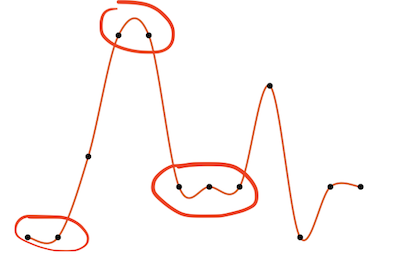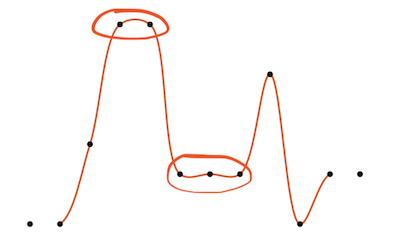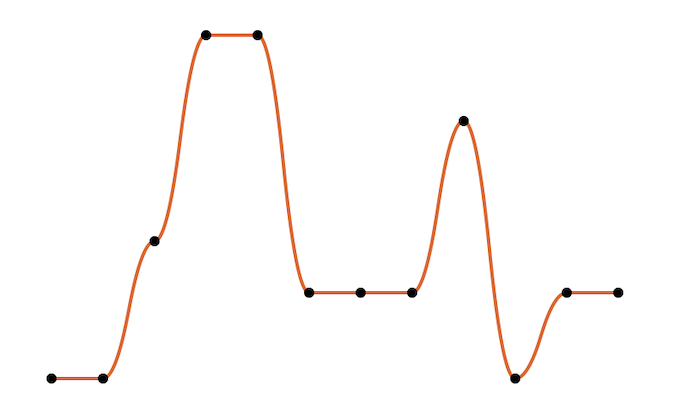# Drawing Smooth 2D Graphs using UIBezierPath

We have been working on multiple projects that required drawing 2D line graphs given a set of points. UIKit gives us the versatile `UIBezierPath`, which allows us to create a straight line graph by appending segments using `addLineToPath` for each point in our array. Easy, but not really pretty.

There are two different kinds of curves in `UIBezierPath`: quadratic curves with a single control point (`addQuadCurveToPoint`) and cubic curves with two control points (`addCurveToPoint`). Calculating the control points is up to you and unfortunately `UIKit` does not come with any ready-to-use implementations of interpolating curves.

Two of the most commonly used techniques are Hermite and Catmull-Rom splines. Details can be found in an in-depth article by John Fisher and a blog post by Ramsundar Shandilya.

However, while great for arbitrary points, both have issues when being used for 2D graphs. Looking at the following screenshots you can see that they extend the curve above the actual maximum and below the minimum y-values. Additionally, even though the three values in the middle section are equal, the graph indicates otherwise.

HermiteCatmull-Rom### Our Result

In our use-case, the above solutions were not acceptable as they may lead to wrong conclusions drawn by the user. To achieve our required results (= a smooth graph that respects global and local maxima and minima and passes through each point), we used quadratic curves. In order for the curve to go through every point, we have to divide each segment into two curves and calculate the control point for each part. Less than 40 lines of code later, we have a nice extension of `UIBezierPath` with exactly the desired results:`````` 1 2 3 4 5 6 7 8 9 10 11 12 13 14 15 16 17 18 19 20 21 22 23 24 25 26 27 28 29 30 31 32 33 34 35 36 37 38 39 40 `````` ``````public extension UIBezierPath { public convenience init?(quadCurve points: [CGPoint]) { guard points.count > 1 else { return nil } self.init() var p1 = points move(to: p1) if points.count == 2 { addLine(to: points) } for i in 0.. CGPoint { return CGPoint(x: (p1.x + p2.x) / 2, y: (p1.y + p2.y) / 2) } private func controlPoint(p1: CGPoint, p2: CGPoint) -> CGPoint { var controlPoint = midPoint(p1: p1, p2: p2) let diffY = abs(p2.y - controlPoint.y) if p1.y < p2.y { controlPoint.y += diffY } else if p1.y > p2.y { controlPoint.y -= diffY } return controlPoint } }``````
Gist

We draw the `UIBezierPath` using `CoreGraphics` and overriding `draw(rect:)` of our UIView subclass, but you could also use a `CAShapeLayer` and even animate the drawing of the path.

This implementation is part of our `SimpleCharts` framework, which we hope to open source in the near future.Looking for a tool to manage all your software translations in one single place?
Checkout texterify.com the new localization and translation management platform.